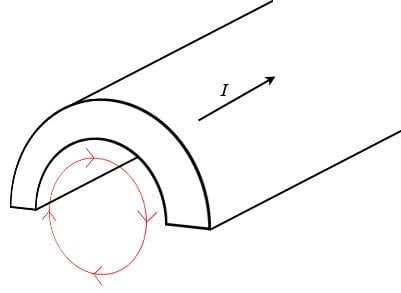# Magnetic field inside hole in half a wire

Nick89
This is not really a homework problem, just something that struck me as weird while revising for an exam...

## Homework Statement

Consider an infinitely long straigt wire with an also infinitely long hole cut out of it.
Then imagine cutting the wire in half, as in the following picture:The 'wire' carries a current I along it's axis.

Now, according to Ampere's law for magnetic fields (see below) there should be no magnetic field inside the hole, right?

Why is this?

I can imagine it if the wire was not cut in half (just a wire with a hole in it) since than the field would cancel itself out (top side cancels out lower side, left side cancels out right side, and so on for every 'pair' of sides).

Now however, that is not the case... So how come there is still no magnetic field in the hole? I just can't imagine this, I think it's weird...

Am I not seeing something obvious maybe?

## Homework Equations

Ampere's law:
$$\oint \vec{B} \cdot \vec{dl} = \mu_0 I_{\text{enclosed}}$$

## The Attempt at a Solution

Using Ampere's law as stated above, with the integral path shown in red in the picture, I find the enclosed current to be 0, resulting in a magnetic field B of also 0...

Is this right, or am I doing something horribly wrong?!

Nick89
Alright I think I figured it out... It would really help me if someone could verify this for me though please!

In the case of a 'normal' wire (without any hole and not cut in half) the magnetic field would be in a circle around the wire's axis. That's why you use a circular integral path when using Ampere's law. In other words, the path you chose has to 'agree' with the direction of the field you're trying to calculate.

Now however, the magnetic field is probably no longer circeling around the axis (at least not uniformly) so the integral path does not agree with the direction of the magnetic field and I cannot use this method.

Am I correct in saying this? Should I use Biot-Savart's law for example instead?

(Note, I'm not trying to actually calculate the field, not for now at least. All I'm trying to do is understand why Ampere's law gives me a 0 magnetic field while my intuition says that can't be true.)

kamerling
for ANY wire, if you integrate around a path that does not go round the wire, you will get 0. That doesn't mean there is no magnetic field.

Nick89
When you take the circular path at a distance from the wire you also get 0 which is obviously wrong...

But, does that mean my last statement is correct? Must the path 'agree' with the direction of B for ampere's law to hold?

In other words, what are the conditions the path must fullfil for ampere's law to be valid?

Last edited:
kamerling
Ampere's law is always valid. If the path doesn't go round the wire then $$\oint \vec{B} \cdot \vec{dl} = \mu_0 I_{\text{enclosed}} = 0$$

Nick89
Yeah I understand that.

I think I got it now. If $$\oint \vec{B} \cdot \vec{dl} = 0$$ that does not imply that B is 0, right? It could also be that the closed loop integral dl is 0 (which in this case it is since the point you are calculating from (the wire) is not inside the path).

Correct?

kamerling
Yeah I understand that.

I think I got it now. If $$\oint \vec{B} \cdot \vec{dl} = 0$$ that does not imply that B is 0, right? It could also be that the closed loop integral dl is 0 (which in this case it is since the point you are calculating from (the wire) is not inside the path).

Correct?
yes indeed.